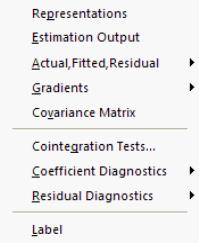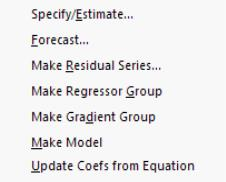User’s Guide : Advanced Single Equation Analysis : Cointegrating Regression : Working with an Equation

Working with an Equation
Once you estimate your equation, EViews offers a variety of views and procedures for examining the properties of the equation, testing, forecasting, and generating new data. For the most part, these views and procedures are a subset of those available in other estimation settings such as least squares estimation. (The one new view, for cointegration testing, is described in depth in “Testing for Cointegration”.) In some cases there have been modifications to account for the nature of cointegrating regression.
ViewsFor the most part, the views of a cointegrating equation require little discussion. For example, the Representations view offers text descriptions of the estimated cointegrating equation, the Covariance Matrix displays the coefficient covariance, and the Residual Diagnostics (Correlogram - Q-statistics, Correlogram Squared Residuals, Histogram - Normality Test) offer statistics based on pooled residuals. That said, a few comments about the construction of these views are in order.
First, the Representations and Covariance Matrix views of an equation only show results for the cointegrating equation and the long-run coefficients. In particular, the short-run dynamics included in a DOLS equation are not incorporated into the equation. Similarly, Coefficient Diagnostics and Gradients views do not include any of the short-run coefficients.
Second, the computation of the residuals used in the Actual, Fitted, Residual views and the Residual Diagnostics views differs depending on the estimation method. For FMOLS and CCR, the residuals are derived simply by substituting the estimated coefficients into the cointegrating equation and computing the residuals. The values are not based on the transformed data. For DOLS, the residuals from the cointegrating equation are adjusted for the estimated short-run dynamics. In all cases, the test statistics results in the Residual Diagnostics should only be viewed is illustrative as they are not supported by asymptotic theory.
Note that standardized residuals are simply the residuals divided through by the long-run variance estimate.
The Gradient (score) views are based on the moment conditions implied by the particular estimation method. For FMOLS and CCR, these moment conditions are based on the transformed data (see Equation (28.25) for the expression for FMOLS scores). For DOLS, these values are simply proportional (-2 times) to the residuals times the regressors.
ProceduresThe procs for an equation estimated using cointegrating regression are virtually identical to those found in least squares estimation.
Most of the relevant issues were discussed previously (e.g., construction of residuals and gradients), however you should also note that forecasts constructed using the Forecast... procedure and models created using Make Model procedure follow the Representations view in omitting DOLS short-run dynamics. Furthermore, the forecast standard errors generated by the Forecast... proc and from solving models created using the Make Model... proc both employ the “S.E. of the regression” reported in the estimation output. This may not be appropriate.
Data Members
The summary statistics results in the bottom of the equation output may be accessed using data member functions (see “Equation Data Members” for a list of common data members). For equations estimated using DOLS (with default standard errors), FMOLS, or CCR, EViews computes an estimate of the long-run variance of the residuals. This statistic may be accessed using the @lrvar member function, so that if you have an equation named FMOLS,
scalar mylrvar = fmols.@lrvar
will store the desired value in the scalar MYLRVAR.